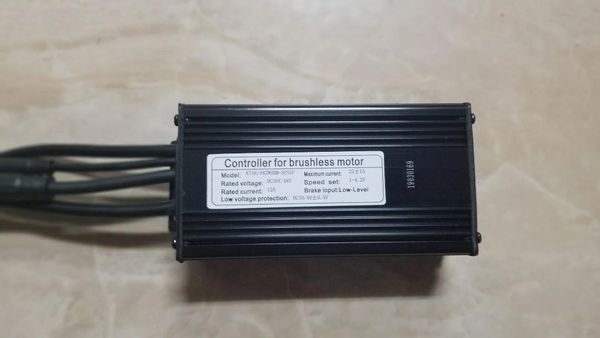# 25a Controller for Rad Mini

\$78.99

Our new upgrade 25 controller for the Rad Mini will give you a 50% power increase from stock! It is plug and play to the Rad Mini and other Rad models with tail light features.

This 25a controller has to be use with our KT-LCD8H display or KT-LCD3/usb display. You can NOT use the Rad's display with our controllers and vice versa. Links to our LCD's are below:

Video of the 25a controller & LCD install

Use these LCD settings for the Rad bike and watch the video link below on how to get into the settings.

P1 = 100

P2 = 5

P3 = 0

P4 = 0

P5 = 15

C1 = 3

C2 = 0

C3 = 8

C4 = 3

C5 = 10

C6 = 3

C7 = 1

C8 = 0

C9 = 0

C10 = n

C11 = 0

C12 = 4

C13 = 0

C14 = 2